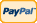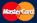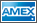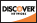•Electronics, electrical engineering
•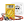Engineering physics
•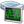Generators
•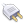Interfaces
•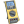Multimeters
•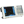Oscilloscopes
•Persona
•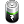Power supplies
•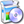Software
•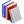Standards
•Thesaurus
•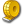Units of measurement
Filter by first letter
A
B
C
D
E
F
G
H
I
J
K
L
M
N
O
P
Q
R
S
T
U
V
W
X
Y
Z
+
-

# Force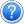In physics, a force is any influence that causes an object to undergo a certain change, either concerning its movement, direction, or geometrical construction. It is measured with the SI unit of newtons and represented by the symbol F. In other words, a force is that which can cause an object with mass to change its velocity (which includes to begin moving from a state of rest), i.e., to accelerate, or which can cause a flexible object to deform. Force can also be described by intuitive concepts such as a push or pull. A force has both magnitude and direction, making it a vector quantity.

The original form of Newton's second law states that the net force acting upon an object is equal to the rate at which its momentum changes with time. If the mass of the object is constant, this law implies that the acceleration of an object is directly proportional to the net force acting on the object, is in the direction of the net force, and is inversely proportional the mass of the object. As a formula, this is expressed as: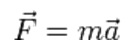where the arrows imply a vector quantity possessing both magnitude and direction.

Related concepts to force include: thrust, which increases the velocity of an object; drag, which decreases the velocity of an object; and torque which produces changes in rotational speed of an object. Forces which do not act uniformly on all parts of a body will also cause mechanical stresses, a technical term for influences which cause deformation of matter. While mechanical stress can remain embedded in a solid object, gradually deforming it, mechanical stress in a fluid determines changes in its pressure and volume.

www.wikipedia.org

Back to the list

Units Converter
• How it Works
• Company News
• Industry News & Events
• Measurement Fun Facts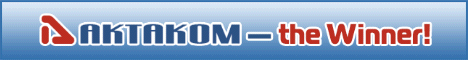Site map|Privacy policy|Terms of Use & Store Policies|How to Buy|Shipping|Payment|© T&M Atlantic, Inc., 2010-2022